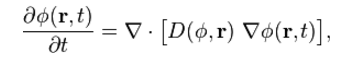# Diffusion formula

Diffusion is the net movement of molecules or atoms from a region of high concentration to lower concentration. It can also be referred to movement of substances towards the lower concentration. The diffusion equation is the partial differentiation equation which indicates dynamics in a material which undergoes diffusion. It also describes processes of exhibiting diffusive behaviour. The diffusion equation is

∂ψ/∂t = 2ψ

The diffusion equation can also be written asWhere

ϕ(r, t) is the density of the diffusing material

D(ϕ, r) is the collective diffusion coefficient

∇ shows the vector differential operator del

If the diffusion coefficient depends on density, then the equation is nonlinear, else it’s linear.

## Solved Examples

Example 1

Solve the diffusion equation ∂ψ / ∂t = kΔ2ψ with the u(x, 0) = f(x), u(0,t) = 0 and u(L, t) = 0

f(X) = 6 sin(πx / L)

Solution

Given equation is

F(x) = 6 sin (πx / L)

U(x, 0) = F(x), u(0,t) = 0 and u(L, t) = 0

Let n = 1

B1 = 6

Using formula,

Un(x, t) = Bn sin(nπx / L) e-k(nπ / L)2 t

U(x, t)  = 6 sin (πx / L) e –k(π / L)2 t

Example 2

Solve the diffusion equation

∂ψ / ∂t = kΔ2ψ with u(x, 0) = f(x), u(0, t) = 0 and u(L, t) = 0

F(x) = 12 sin (π x / L)

Solution

u(x, 0) = f(x), u(0, t) = 0 and u(L, t) = 0

Let n = 1

B1 = 12

From the formula,

Un(x, t) = Bn sin (n π x / L) e –k(2 π / L)2 t

U(x, t) = 12 sin(π x / L) e –k(π/L)2 t